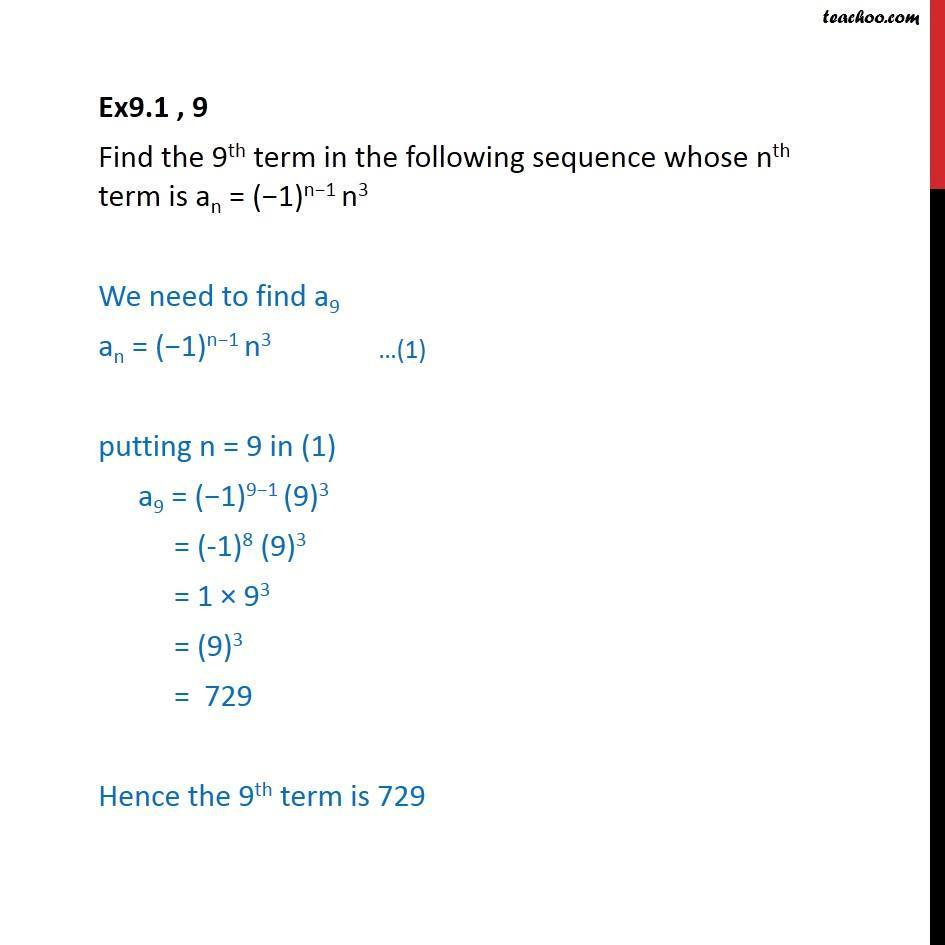Ex 8.1

Chapter 8 Class 11 Sequences and Series
Serial order wiseLearn in your speed, with individual attention - Teachoo Maths 1-on-1 Class

### Transcript

Ex9.1 , 9 Find the 9th term in the following sequence whose nth term is an =" (−1)n−1 n3" We need to find a9 an =" (−1)n−1 n3" putting n = 9 in (1) a9 =" (−1)9−1 (9)3 " = (-1)8 "(9)3" = 1 × 93 = "(9)3" = 729 Hence the 9th term is 729